#### Computing backprojections

Many algorithms have been developed to compute backprojections. The first algorithm was given in [311,312]. Assume that the goal region is one or more segments contained in edges of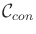. The algorithm proceeds for a fixed motion command,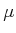, which is based on a direction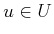as follows:

1. Mark every obstacle vertex at which sticking is possible. Also mark any point on the boundary of the goal region if it is possible to slide away from the goal.
2. For every marked vertex, extend two rays with directions based on the maximum possible deviations allowed by nature when executing. This inverts the cone shown in Figure 12.45a. The extended rays are shown in Figure 12.48 for the frictionless case (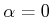).
3. Starting at every goal edge, trace out the boundary of the backprojection region. Every edge encountered defines a half-plane of configurations from which the robot is guaranteed to move into. In Figure 12.48, this corresponds to being below a ray. When tracing out the backprojection boundary, the direction at each intersection vertex is determined based on including the points in the half-plane.
The resulting backprojection is shown in Figure 12.49. A more general algorithm that applies to goal regions that include polygonal regions inwas given in  (some details are also covered in ). It uses the plane-sweep principle (presented in Section 6.2.2) to yield an algorithm that computes the backprojection in time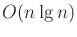, in whichis the number of edges used to define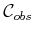. The backprojection itself has no more than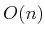edges. Algorithms for computing nondirectional backprojections are given in [140,283]. One difficulty in this case is that the backprojection boundary may be quite complicated. An incremental algorithm for computing a nondirectional backprojection of size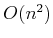in time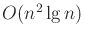is given in .

Once an algorithm that computes backprojections has been obtained, it needs to be adapted to compute preimages. Using the sensing model shown in Figure 12.45b, a preimage can be obtained by shrinking the subgoal region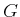. Let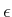denote the radius of the ball in Figure 12.45b. Let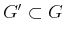denote a subset of the subgoal in which a strip of thicknesshas been removed. If the sensor returns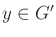, then it is guaranteed that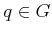. This yields a method of obtaining preimages by shrinking the subgoals. Ifis too large, however, this may fail to yield a successful plan, even though one exists.

The high-level plan can be found by performing a backward search that computes backprojections from the goal region (reduced by). There is still the difficulty of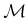being too large, which controls the branching factor in the search. One possibility is to compute nondirectional backprojections. Another possibility is to discretize. For example, in [588,590],is reduced to four principle directions, and plans are computed for complicated environments by using sticking edges as subgoals. Using discretization, however, it becomes more difficult to ensure the completeness of the planning algorithm.

The preimage planning framework may seem to apply only to a very specific model, but it can be extended and adapted to a much more general setting. It was extended to semi-algebraic obstacle models in , which gives a planning method that runs in time doubly exponential in the C-space dimension (based on cylindrical algebraic decomposition, which was covered in Section 6.4.2). In , probabilistic backprojections were introduced by assigning a uniform probability density function to the nature action spaces considered in this section. This was in turn generalized further to define backprojections and preimages as the level sets of optimal cost-to-go functions in [597,605]. Dynamic programming methods can then be applied to compute plans.

Steven M LaValle 2020-08-14